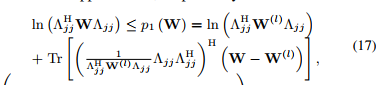I get the following error in a small probability when using rel_entr_quad,

And the corresponding code is as follows

And the expression of Equation_first_1 isBy the way, when I don’t add the eps in the code, this problem happens more frequently. I appreciate any help!

That is happening due to the 2nd argument of `rel_entr_quad`, i.e., the argument of `log`, being negative, even if just barely so. You need to ensure that argument is nonnegative.

I can’t be more specific because I don’t know which of the things in that argument are CVX variables or expressions, as opposed to input data. If `W_1` is a matrix variable which is constrained to be semidefinite, that argument should in theory be nonnegative. If `W_1` is input data and is semidefinite, that should also be nonnegative. It may be that a simple fix to either of those situations is to use a larger number instead of `eps`, for instance, `1e-8`. If `W_1` is not semidefinite, there is no reason that argument would have to be nonnegative.

BTW, if you have access to Mosek, it is better to Mosek as solver, combined with use of `log(argument)`, rather than using `-rel_entr_quad(1,argument)`